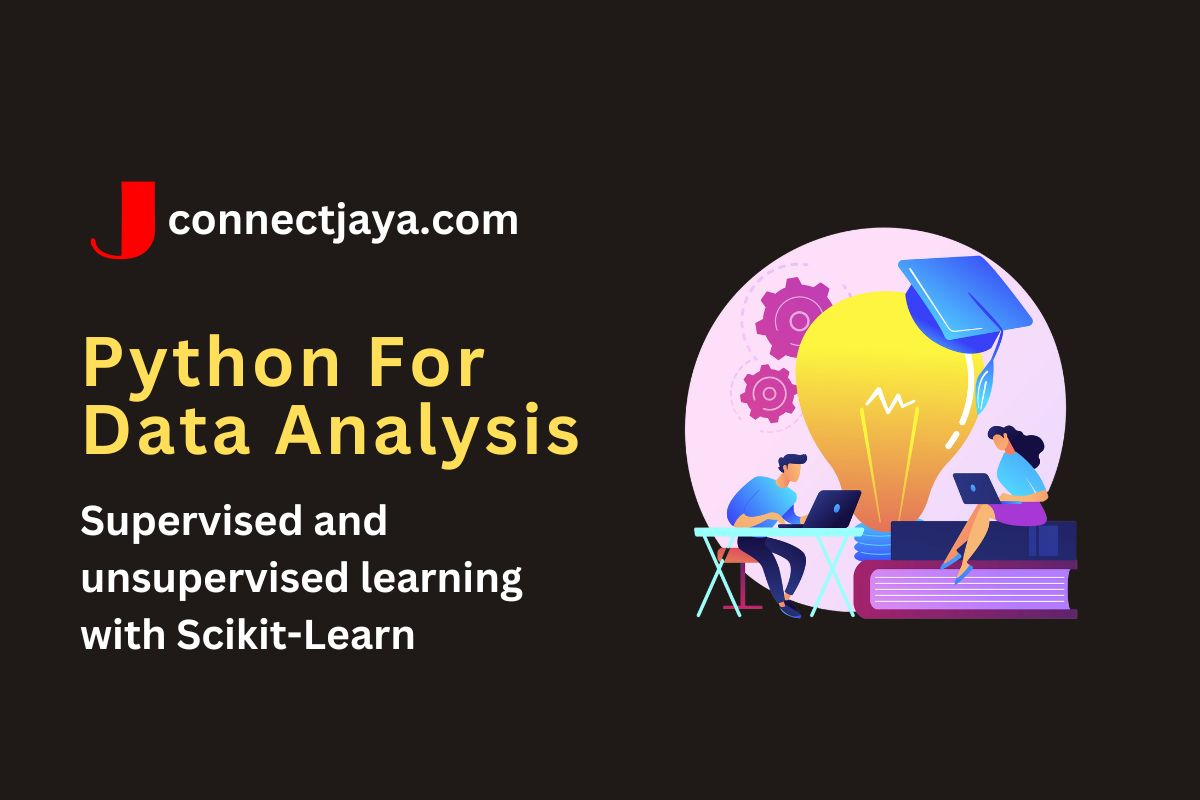## Supervised and unsupervised learning with Scikit-Learn

Scikit-Learn supports both supervised and unsupervised learning, which are two of the main categories of machine learning.

Supervised learning involves building a model to predict an output variable (also known as the response variable or dependent variable) based on one or more input variables (also known as predictors or independent variables), using a labeled dataset. Scikit-Learn provides a wide range of supervised learning algorithms, including.

• Linear regression: Used to predict a continuous output variable.
• Logistic regression: Used to predict a binary or categorical output variable.
• Decision trees: Used to predict a categorical or continuous output variable.
• Random forests: An ensemble method that combines multiple decision trees.
• Support vector machines (SVMs): Used to predict a categorical or continuous output variable.
• Naive Bayes: Used to predict a categorical output variable.
• Neural networks: Used to predict a categorical or continuous output variable.

### Here’s an example of how to use Scikit-Learn to build a logistic regression model for the Iris dataset.

from sklearn.datasets import load_iris
from sklearn.linear_model import LogisticRegression
from sklearn.model_selection import train_test_split
from sklearn.metrics import accuracy_score

# Split the data into training and testing sets
X_train, X_test, y_train, y_test = train_test_split(
iris.data, iris.target, test_size=0.2, random_state=42)

# Create a logistic regression model and fit the training data
model = LogisticRegression()
model.fit(X_train, y_train)

# Make predictions on the testing data
y_pred = model.predict(X_test)

# Calculate the accuracy of the model
accuracy = accuracy_score(y_test, y_pred)

print("Accuracy:", accuracy)


In this example, we first load the Iris dataset using Scikit-Learn’s built-in load_iris() function. We then split the data into training and testing sets using the train_test_split() function. Next, we create a logistic regression model using the LogisticRegression class and fit the training data using the fit() method. Finally, we make predictions on the testing data using the predict() method and calculate the accuracy of the model using the accuracy_score() function.

Unsupervised learning, on the other hand, involves discovering patterns and relationships in a dataset without a specific output variable, using an unlabeled dataset. Scikit-Learn provides a wide range of unsupervised learning algorithms, including.

• Clustering: Used to group similar data points together.
• Dimensionality reduction: Used to reduce the number of input variables while preserving important information.
• Anomaly detection: Used to identify unusual or anomalous data points.

#### Here’s an example of how to use Scikit-Learn to perform k-means clustering on the Iris dataset.

from sklearn.datasets import load_iris
from sklearn.cluster import KMeans

# Create a k-means clustering model with 3 clusters
model = KMeans(n_clusters=3, random_state=42)

# Fit the model to the data
model.fit(iris.data)

# Get the cluster labels for each data point
labels = model.labels_

print(labels)


In this example, we first load the Iris dataset using Scikit-Learn’s built-in load_iris() function. We then create a k-means clustering model using the KMeans class with 3 clusters and fit the model to the data using the fit() method. Finally, we get the cluster labels for each data point using the labels_ attribute.